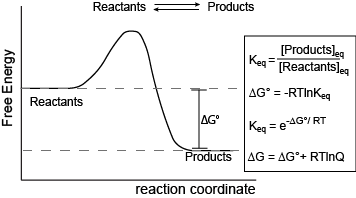7.4: Chemical Equilibrium—Part 2: Free Energy

Chemical Equilibrium—Part 2: Gibbs Energy

In a previous section, we began a description of chemical equilibrium in the context of forward and reverse rates. Three key ideas were presented:

1. At equilibrium, the concentrations of reactants and products in a reversible reaction are not changing in time.
2. A reversible reaction at equilibrium is not staticreactants and products continue to interconvert at equilibrium, but the rates of the forward and reverse reactions are the same.
3. We were NOT going to fall into a common student trap of assuming that chemical equilibrium means that the concentrations of reactants and products are equal at equilibrium.

Here we extend our discussion and put the concept of equilibrium into the context of Gibbs energy, also reinforcing the Energy Story exercise of considering the "Before/Start" and "After/End" states of a reaction (including the inherent passage of time).Figure 1. Reaction coordinate diagram for a generic exergonic reversible reaction. Equations relating Gibbs energy and the equilibrium constant: R = 8.314 J mol-1 K-1 or 0.008314 kJ mol-1 K-1; T is temperature in Kelvin. Attribution: Marc T. Facciotti (original work)

The figure above shows a commonly cited relationship between ∆G° and Keq:

$∆G^o = -RT\ln K_{eq}.$

Here, G° indicates the Gibbs energy under standard conditions (e.g., 1 atmosphere of pressure, 298 K). This equation describes the change in Gibbs energy for reactants converting to products in a reaction that is at equilibrium. The value of ∆G° can therefore be thought of as being intrinsic to the reactants and products themselves. ∆G° is like a potential energy difference between reactants and products. With this concept as a basis, one can also consider a reaction where the "starting" state is somewhere out of equilibrium. In this case, there may be an additional “potential” associated with the out-of-equilibrium starting state. This “added” component contributes to the ∆G of a reaction and can be effectively added to the expression for Gibbs energy as follows:

$∆G = ∆G° + RT\ln Q,$

where $$Q$$ is called the reaction quotient. From the standpoint of BIS2A, we will use a simple (a bit incomplete but functional) definition for

$Q = \dfrac{[Products]_{st}}{[Reactants]_{st}}$

at a defined non-equilibrium condition, st. One can extend this idea and calculate the Gibbs energy difference between two non-equilibrium states, provided they are properly defined and thus compute Gibbs energy changes between specifically defined out-of-equilibrium states. This last point is often relevant in reactions found in biological systems as these reactions are often found in multi-step pathways that effectively keep individual reactions in an out-of-equilibrium state.

This takes us to a point of confusion for some. In many biology books, the discussion of equilibrium includes not only the discussion of forward and reverse reaction rates, but also a statement that ∆G = 0 at equilibrium. This can be confusing because these very discussions often follow discussions of non-zero ∆G° values in the context of equilibrium (∆G° = -RTlnKeq). The nuance to point out is that ∆G° is referring to the Gibbs energy potential inherent in the chemical transformation between reactants and products alone. This is different from considering the progress of the reaction from an out-of-equilibrium state that is described by

$∆G = ∆G^o + RT \ln Q.$

This expression can be expanded as follows:

$∆G = -RT\ln K_{eq} + RT\ln Q$

to bring the nuance into clearer focus. In this case note that as Q approaches Keq that the reaction ∆G becomes closer to zero, ultimately reaching zero when Q = Keq. This means that the Gibbs energy of the reaction (∆G) reaches zero at equilibrium not that the potential difference between substrates and products (∆G°) reaches zero.